SEARCH HOMEMath Central Quandaries & QueriesQuestion from Art, a student: what percent of 85 is 17?Hi Art.

I will show you how to solve this with another set of numbers. What percent of 64 is 16?

The important idea is that the ratios (fractions) are the same. A percentage is just a fraction. It literally means "per hundred". So 70% is 70 per 100 which is 70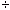100.

With algebra we use a letter to represent an unknown value.

Let P be the percentage that 16 is of 64.

Then we can write this as two fractions that equal each other: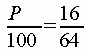Now we want to "solve" this equation for P. That means we must multiply both sides of the equation by 100: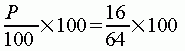This lets us cancel out the 100 in the denominator with the 100 we multiplied both sides of the equation by: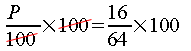Finally, we can divide 16 by 64 and then multiply by 100 on the right hand side to find the answer.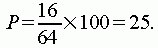So in this case 16 is 25% of 64.

Your question can be solved exactly the same way, Art.

Hope this helps,
Stephen La Rocque.Math Central is supported by the University of Regina and The Pacific Institute for the Mathematical Sciences.